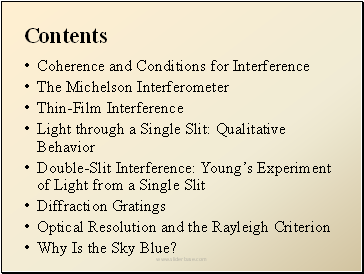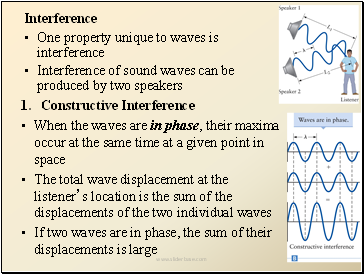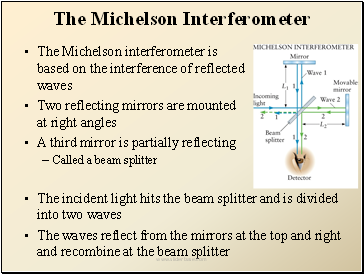# Wave OpticsPage 1

#### WATCH ALL SLIDES

Slide 1Contents

Coherence and Conditions for Interference

The Michelson Interferometer

Thin-Film Interference

Light through a Single Slit: Qualitative Behavior

Double-Slit Interference: Young’s Experiment of Light from a Single Slit

Diffraction Gratings

Optical Resolution and the Rayleigh Criterion

Why Is the Sky Blue?

Slide 2## Coherence and Conditions for Interference

Wave Optics

The field of wave optics studies the properties of light that depend on its wave nature

Originally light was thought to be a particle and that model successfully explained the phenomena discussed in geometric optics

Maxwell’s theory of electromagnetism convinced physicists that light was a wave

When discussing image characteristics over distances much greater than the wavelength, geometric optics is extremely accurate

When dealing with sizes comparable to or smaller than the wavelength, wave optics is required

Slide 3Interference

One property unique to waves is interference

Interference of sound waves can be produced by two speakers

Constructive Interference

When the waves are in phase, their maxima occur at the same time at a given point in space

The total wave displacement at the listener’s location is the sum of the displacements of the two individual waves

If two waves are in phase, the sum of their displacements is large

Slide 4Destructive Interference

The maximum of one wave can coincide with the minimum of the other wave

These waves are out of phase

The interference is destructive when the waves are out of phase

If the waves are 180° out of phase, the sum of the displacements of the two waves is zero

Slide 5## General conditions for interference.

Two waves can interfere if all the following conditions are met.

1. Two or more interfering waves travel through different regions of space over at least part of their propagation from source to destination.

2. The waves are brought together at a common point.

3. The waves must have the same frequency and must also have a fixed phase relationship. Thus, over a given distance or time interval the phase difference between the waves remains constant. Such waves are called coherent.

Slide 6## The Michelson Interferometer

The Michelson interferometer is based on the interference of reflected waves

Two reflecting mirrors are mounted at right angles

Go to page:
1  2  3  4  5  6